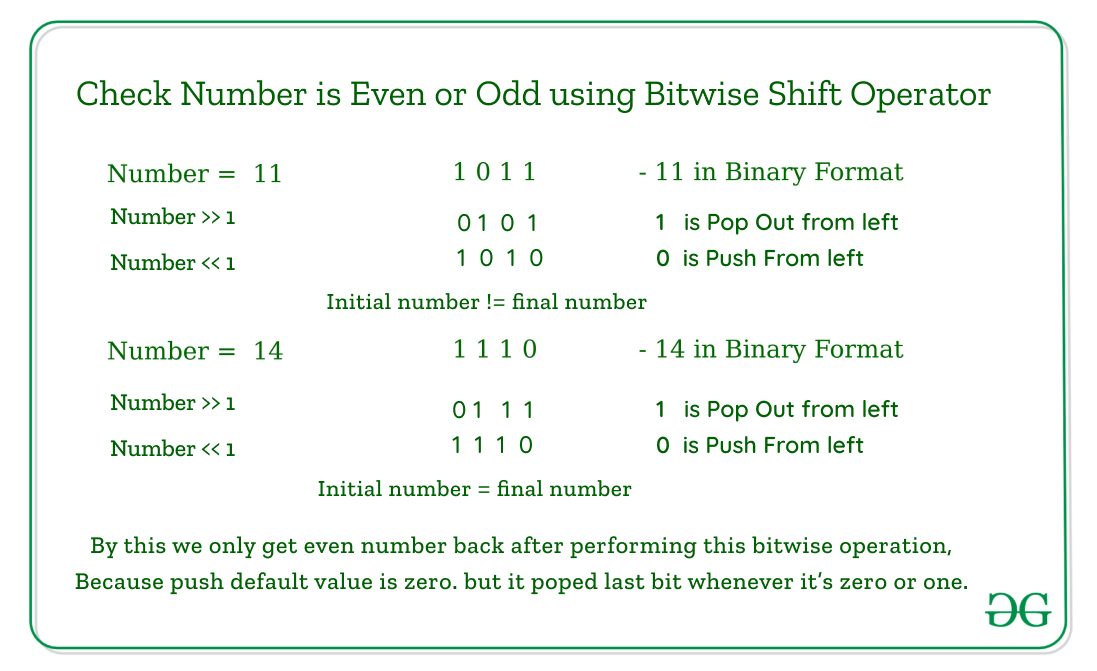Open in App
Not now

# C program to check whether a given number is even or odd

• Difficulty Level : Expert
• Last Updated : 04 Dec, 2022

Given an integer N, the task is to check if the given number N is even or odd. If it is found to be even, then print “Even”. Otherwise, print “Odd”. Examples:

Input: N = 2
Output: Even Input: N = 5
Output: Odd

## Recommended: Please try your approach on {IDE} first, before moving on to the solution.

Method 1: The simplest approach is to check if the remainder obtained after dividing the given number N by 2 is 0 or 1. If the remainder is 0, then print “Even”. Otherwise, print “Odd”. Below is the implementation of the above approach:

## C

 `// C program for the above approach``#include ` `// Function to check if a``// number is even or odd``void` `checkEvenOdd(``int` `N)``{``    ``// Find remainder``    ``int` `r = N % 2;` `    ``// Condition for even``    ``if` `(r == 0) {``        ``printf``(``"Even"``);``    ``}` `    ``// Otherwise``    ``else` `{``        ``printf``(``"Odd"``);``    ``}``}` `// Driver Code``int` `main()``{``    ``// Given number N``    ``int` `N = 101;` `    ``// Function Call``    ``checkEvenOdd(N);` `    ``return` `0;``}`

Output:

`Odd`

Time Complexity: O(1)
Auxiliary Space: O(1)

Method 2: Another approach is to use Bitwise Operators. The idea is to check whether the last bit of the given number N is 1 or not. To check whether the last bit is 1 find the value of (N & 1). If the result is 1, then print “Odd”. Otherwise, print “Even”. Below is the illustration for N = 5:

N = 5.
Binary representation of 5 is 00000101
Binary representation of 1 is 00000001
——————————————————————-
The value of Bitwise AND is 00000001 Since the result is 1. Therefore, the number N = 5 is odd.

Below is the implementation of the above approach:

## C

 `// C program for the above approach` `#include ` `// Function to check if a``// number is even or odd``void` `checkEvenOdd(``int` `N)``{``    ``// If N & 1 is true``    ``if` `(N & 1) {``        ``printf``(``"Odd"``);``    ``}` `    ``// Otherwise``    ``else` `{``        ``printf``(``"Even"``);``    ``}``}` `// Driver Code``int` `main()``{``    ``// Given number N``    ``int` `N = 101;` `    ``// Function Call``    ``checkEvenOdd(N);` `    ``return` `0;``}`

Output:

`Odd`

Time Complexity: O(1)
Auxiliary Space: O(1)

Method 3: The idea is to initialize an integer variable var as 1 and change it from 1 to 0 and vice-versa alternately, N times. If var is equal to 1 after N operations, print “Even”. Otherwise, print “Odd”. Below is the implementation of the above approach:

## C

 `// C program for the above approach``#include ` `// Function to check a number is``// even or odd``void` `checkEvenOdd(``int` `N)``{``    ``// Initialise a variable var``    ``int` `var = 1;` `    ``// Iterate till N``    ``for` `(``int` `i = 1; i <= N; i++) {` `        ``// Subtract var from 1``        ``var = 1 - var;``    ``}` `    ``// Condition for even``    ``if` `(var == 1) {``        ``printf``(``"Even"``);``    ``}` `    ``// Otherwise``    ``else` `{``        ``printf``(``"Odd"``);``    ``}``}` `// Driver Code``int` `main()``{``    ``// Given number N``    ``int` `N = 101;` `    ``// Function Call``    ``checkEvenOdd(N);` `    ``return` `0;``}`

Output:

`Odd`

Time Complexity: O(1)
Auxiliary Space: O(1)

Method 4: Another approach is by using bitwise left-shift and right-shift operators. The logic behind this implementation is about regenerating the value after the right shift and left shift. We all know even numbers have zero as the last bit and odd have one as the last bit. When we bitwise right shift any number then the last bit of the number piped out whenever it is even or odd. Next, we did a bitwise left shift, then our bit shifted by one. Now last bit placed is empty which is by default filled by a zero. During all these odd numbers changed their value but even remains the same. That’s how by comparing the initial and final value we decide number is even or odd.below is the implementation of the above logic.

## C

 `#include ` `int` `main() {` `  ``int` `a = 7 ;``   ``// To take input from user, prefer below code``   ``// int a ;``   ``// printf("Enter an number: \n");``   ``// scanf("%d",&a);``  ` `    ``if``(a == ( a>>1 ) << 1){``        ``printf``(``"Number %d is even.\n"``,a);``     ``}``    ``else``{``         ``printf``(``"Number %d is not even.\n"``,a);``     ``}` `   ``// below is short hand``   ``// a==(a>>1)<<1? printf("Number %d is even.\n",a):printf("Number %d is odd.\n",a);``  ` `    ``return` `0;``}`

`Output: Number 7 is odd.`

My Personal Notes arrow_drop_up